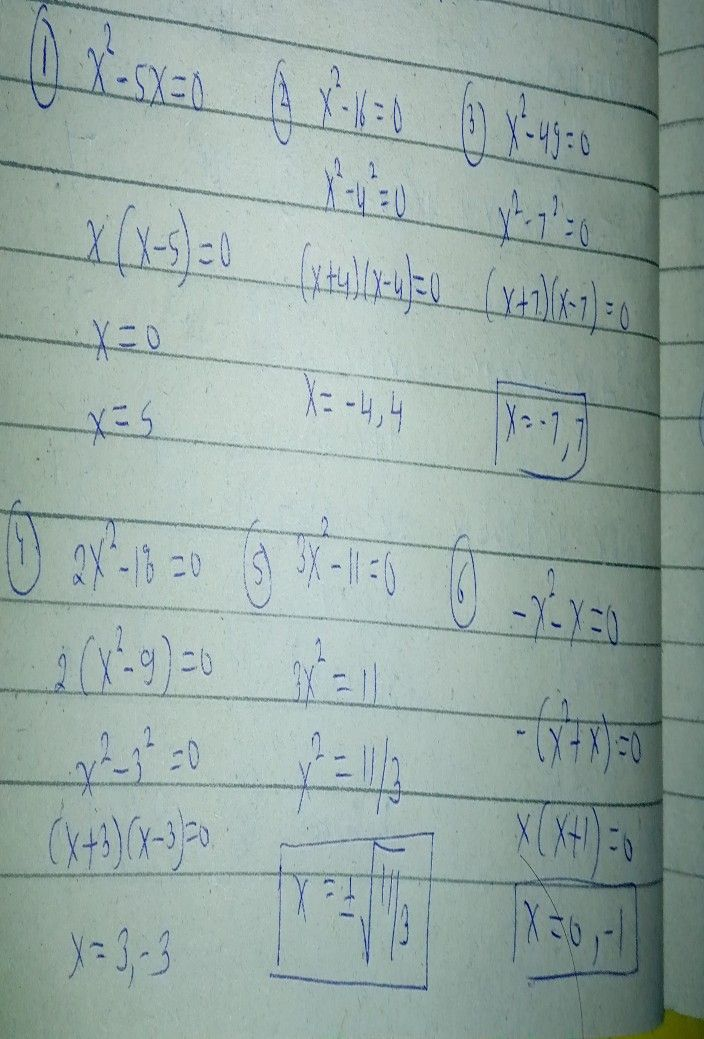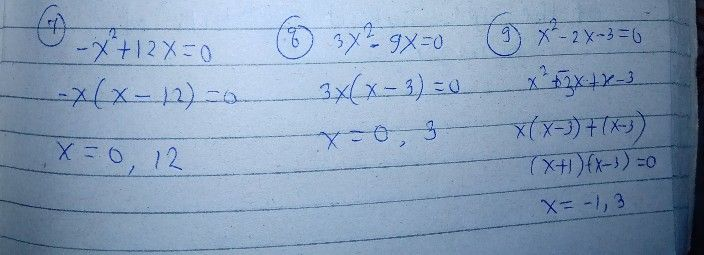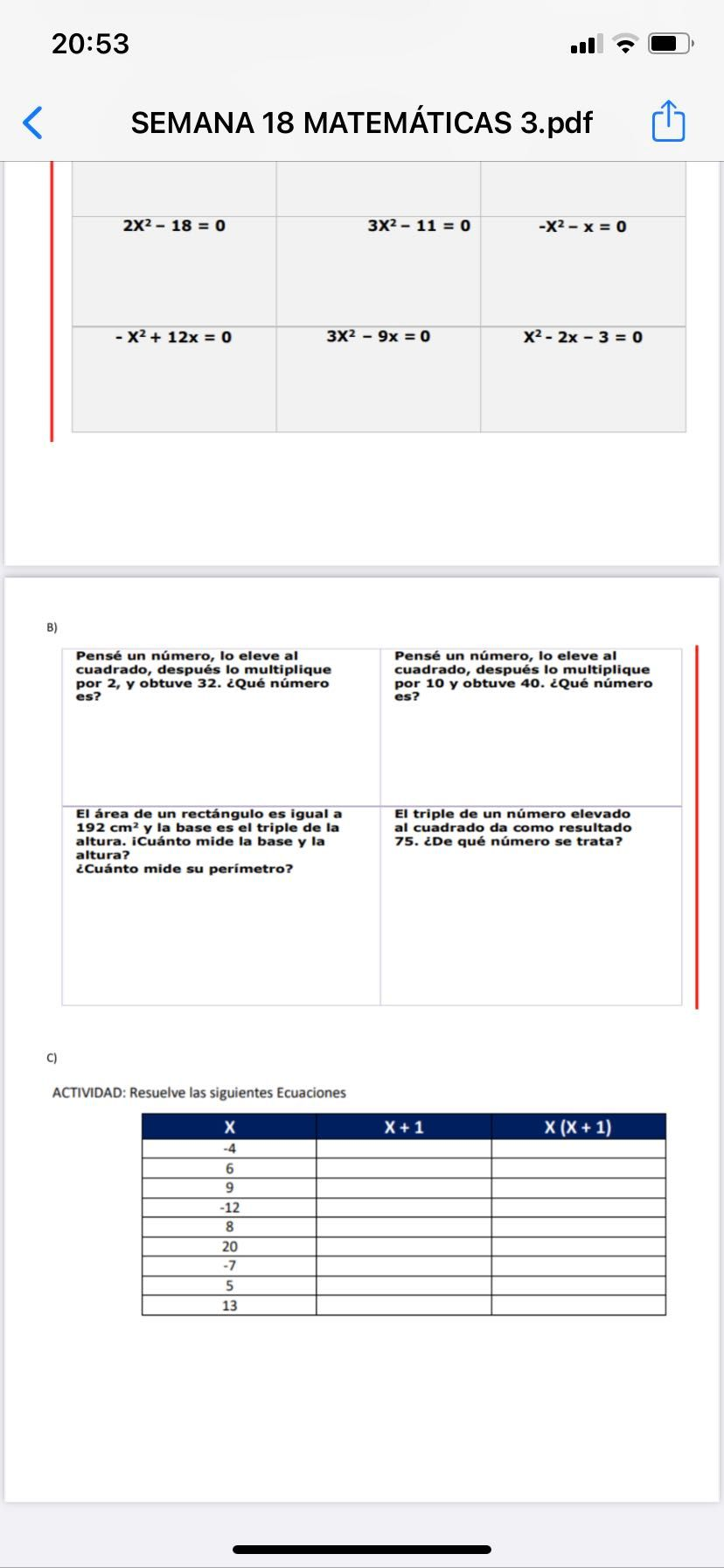Symbol
Problem$1.=$ Resuelve las siguientes Ecuaciones. $x^{2}-5x=0$ $x^{2-16}$ $=$ $0$ $x^{2}=$ $49=0$ $2x2-18=0$ $3x2-11=0$ $-x2-x=0$ $-x^{2}+12x=0$ $3x2-9x=0$ $x^{2}-2x-3=0$
Other
SolutionQanda teacher - Arushithanks for asking a good question
any doubt so pls ask me to know
kindly evaluate ans with gift coins and Rating 5 star
thanksStudent
GraciasQanda teacher - Arushi
We are only one question allow at time by qanda policy
if you have more questions so pls ask on preferred match on my profile
ohk
thanks for asking a good question